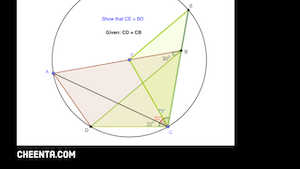How Cheenta works to ensure student success?
Explore the Back-Story

# INMO 2021 Problem 5 - Solution and DiscussionIn a convex quadrilateral $A B C D, \angle A B D=30^{\circ}, \angle B C A=75^{\circ}, \angle A C D=25^{\circ}$ and
$C D=C B$. Extend $C B$ to meet the circumcircle of triangle $D A C$ at $E$. Prove that $C E=B D .$

###### Hint and Solution for INMO 2021 Problem 5

In a convex quadrilateral $A B C D, \angle A B D=30^{\circ}, \angle B C A=75^{\circ}, \angle A C D=25^{\circ}$ and
$C D=C B$. Extend $C B$ to meet the circumcircle of triangle $D A C$ at $E$. Prove that $C E=B D .$

###### Hint and Solution for INMO 2021 Problem 5

This site uses Akismet to reduce spam. Learn how your comment data is processed.

### Knowledge Partner